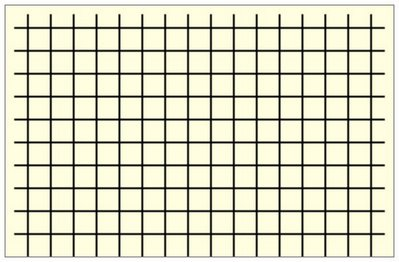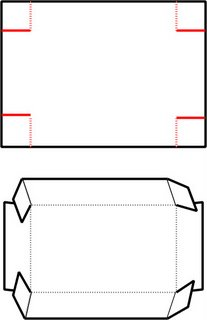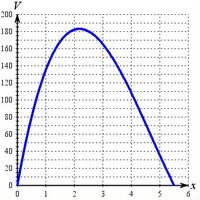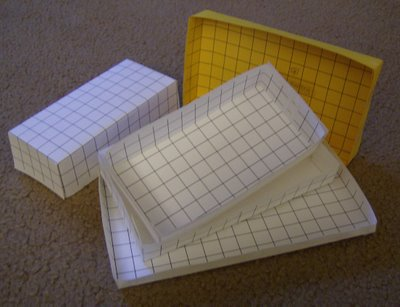Saturday, December 23, 2006

Thinking inside the boxA hands-on math problem

Everyone who's taken elementary calculus has met the box problem: Suppose you have a rectangular piece of material. If you cut squares out of the four corners and fold up the sides, you create a box. What is the size of the squares you should cut out if you want the volume of your box to be a maximum?

If the rectangular piece of material is a square, the answer turns out to be the side of each corner square should be one-sixth of the original square's side. If the rectangle is not a square, it gets more interesting.

Since the problem is easy to model and visualize, it's a classroom favorite. You can present the problem to a class in many different ways: computer models, spreadsheet calculations, or stand-and-deliver symbol manipulation. I have come to prefer a very mundane approach that has sparked lots of student participation. You'll need paper (heavier stock is better), scissors, and tape. Yes, we're going to actually build some boxes by hand.

My first step is to print out a bunch of full-page half-inch grids on card stock. You can use the table feature in Word or WordPerfect or a spreadsheet grid to force horizontal and vertical rules spaced half an inch apart. Since printers can't print to the very edge of a page, the grid won't be quite complete. I turn off the outermost borders on the outermost grid boxes and tweak their size in conjunction with the print margins so that the spacing remains half an inch all the way across. Then I cut each 8½ by 11 sheet in two, creating a pair of rectangles marked off in a square grid, each one 11 by 17 (using half-inch units, of course).It's very easy to hand out rectangles, scissors, and tape and tell your students to start cutting, folding, and taping up boxes. However, most of them will immediately pick 2 by 2 or 3 by 3 squares, so you end up with big stacks of duplicates. Here's what I do to avoid this problem:I break the class up into groups of four. Each student has a rectangle and each group has a pair of scissors and a roll of tape. The student to whom I hand the scissors in each group is designated the Recorder and is given a tally sheet on which to enter the results of the group's work. The Recorder gets to make the first box, choosing how big the corner squares should be in his or her box construction. When the Recorder has finished using the scissors, they are handed off to another student in the group, who is not allowed to use the same size cuts as the Recorder. In fact, each student is required to choose cuts of different dimensions, so that the group will produce four distinct boxes.

Here are some of the directions on an instruction sheet that each student has:
When it’s your turn, take your cardboard rectangle, which is 17 units long by 11 units wide, and choose how big a square to cut out of the corners. You cannot choose the same size as anyone else in your group. Don’t actually remove the squares, just make one cut in each corner so that you can fold up the sides and make a rectangular box. Tape the corners.

Write your name on your box, along with the length, width, height, and volume. Make sure the recorder has all of your information.
The pressure to choose different sizes for the square corners produces interesting results. Instead of choosing small integers like 2 or 3, some announce that they will use e or π in addition to more conventional “risky” numbers like 1.75 or 2.5.

The Recorders take their tally sheets to the board and write down the cut sizes, each accompanied by the volume of the resulting box. I plot these on a Cartesian coordinate board at the side of the room. A few minutes are spent tracking down and correcting errant results (usually given away by failing to fall on the curve that is forming on the coordinate board).

The cutting and taping and tallying takes about twenty minutes with a class of thirty. The students soon agree that the 182 cubic units corresponding to a cut of 2 must be the maximum possible volume (after which there's often some grumbling among those who claim they wanted to choose 2 but were prevented from doing so by the rules of the exercise). That sets up phase two of the experiment.

I hand out worksheets on which the groups are to replicate their construction-paper experiments, but this time with x playing the role of the cut. The students collaborate in figuring out that the dimensions of the resulting box would have to be 17 − 2x by 11 − 2x by x, after which they compute and simplify an expression for the volume of the box as a function of x:

V(x) = 4x3 − 56x2 + 187x.Rather to their surprise, as they work through finding the first derivative and setting it equal to zero to find critical values, the students discover that x = 2 is not the value corresponding to a maximum. It turns out to be x = 2.18. (That's an approximation, of course. The exact result contains a radical.) A volume of almost 183 cubic units is actually possible.

The classroom exercise in box construction appeals to me in several ways: There is an apparent result which turns out to be not quite correct. There is a an opportunity to work together in tracking down the exact answer. There is the chance to learn by personal construction and computation how a function can be developed to represent a quantity to be optimized. It's a lot better than following a teacher's scratch marks on a board in front of a class. On top of all that, the materials are cheap and easy to come by.

By the way, even though I've always conducted this exercise with college students, I have found it prudent to distribute round-ended safety scissors. I'm just saying.

You can find a charming Java applet treatment of the box problem in all of its rectangular generality at Alexander Bogomolny's Interactive Mathematics site.Jokermage said...

I was able to create a TI-83 program to solve the general problem. It's a very simple problem if you can multiply polynomials, take simple derivatives and solve the quadratic formula.

Given a retangular paper with length L and width W...
The height of the box will be x.
The length of the box will be (L-2x)
The width of the box will be (W-2x)

Multiplying these together gives a volume equation of V = 4x^3 - 2(L+W)x^2 + (L*W)x
The derivative of this would be V' = 12x^2 - 4(L+W)x + (L*W)
Where the derivative is zero will be the optimal height.

x = (4(L+W) - Sqrt(16(L+W)^2 - 48(L*W))) / 24
Once you solve for x, plug it into the volume equation to calculate the max volume.

So did I get it right?